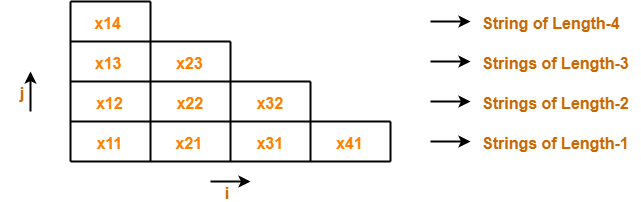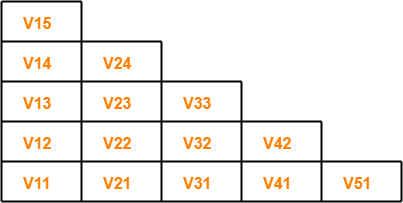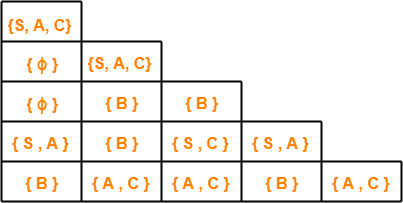## CYK Algorithm-

 CYK Algorithm is a membership algorithm of context free grammar.

• It is used to decide whether a given string belongs to the language of grammar or not.
• It is also known as CKY Algorithm or Cocke-Younger-Kasami Algorithm after its inventors.

## Important Notes-

### Note-02:

• The worst case running time of CYK Algorithm is Θ (n3.|G|).
• Here, n = Length of parsed string and |G| = Size of the CNF Grammar G.

## Algorithm-

```Begin
for ( i = 1 to n do )
Vi1 { A | A → a is a production where ith symbol of x is a }

for ( j = 2 to n do )
for ( i = 1 to n - j + 1 do )
Begin
Vij = ϕ
For k = 1 to j - 1 do
Vij = Vij ∪ { A | A → BC is a production where B is in Vik and C is in V(i + k)(j - k) }
End
End```

Also Read- Algorithm To Check Whether CFL is Empty

## Deciding Membership Using CYK Algorithm-

To decide the membership of any given string, we construct a triangular table where-

• Each row of the table corresponds to one particular length of sub strings.
• Bottom most row corresponds to strings of length-1.
• Second row from bottom corresponds to strings of length-2.
• Third row from bottom corresponds to strings of length-3.
• Top most row from bottom corresponds to the given string of length-n.

## Example-

For a given string “x” of length 4 units, triangular table looks like-We will fill each box of xij with its Vij.

These notations are discussed below.

## Notations Used

### Notation-01: xij

xij represents a sub string of “x” starting from location ‘i’ and has length ‘j’.

Example-

Consider x = abcd is a string, then-

Number of sub strings possible = n(n+1)/2 = 4 x (4+1) / 2 = 10

We have-

• x11 = a
• x21 = b
• x31 = c
• x41 = d
• x12 = ab
• x22 = bc
• x32 = cd
• x13 = abc
• x23 = bcd
• x14 = abcd

### Notation-02: Vij

Vij represents a set of variables in the grammar which can derive the sub string xij.

If the set of variables consists of the start symbol, then it becomes sure-

• Sub string xij can be derived from the given grammar.
• Sub string xij is a member of the language of the given grammar.

Also Read- Algorithm To Check Whether CFL is Finite

## Problem-

For the given grammar, check the acceptance of string w = baaba using CYK Algorithm-

S → AB / BC

A → BA / a

B → CC / b

C → AB / a

## Solution-

First, let us draw the triangular table.

• The given string is x = baaba.
• Length of given string = |x| = 5.
• So, Number of sub strings possible = (5 x 6) / 2 = 15.

So, triangular table looks like-Now, let us find the value of Vij for each cell.

## For Row-1:

### Finding V11

• V11 represents the set of variables deriving x11.
• x11 = b.
• Only variable B derives string “b” in the given grammar.
• Thus, V11 = { B }

### Finding V21

• V21 represents the set of variables deriving x21.
• x21 = a.
• Variables A and C derive string “a” in the given grammar.
• Thus, V21 = { A , C }

### Finding V31

• V31 represents the set of variables deriving x31.
• x31 = a.
• Variables A and C derive string “b” in the given grammar.
• Thus, V31 = { A , C }

### Finding V41

• V41 represents the set of variables deriving x41.
• x41 = b.
• Only variable B derives string “b” in the given grammar.
• Thus, V41 = { B }

### Finding V51

• V51 represents the set of variables deriving x51.
• x51 = a.
• Variables A and C derives string “a” in the given grammar.
• Thus, V51 = { A , C }

## For Row-2-

### RULE

As per the algorithm, to find the value of Vij from 2nd row on wards,

we use the formula-

Vij = Vik V(i+k)(j-k)

where k varies from 1 to j-1

### Finding V12

We have i = 1 , j = 2 , k = 1

Substituting values in the formula, we get-

V12 = V11. V21

V12 = { B } { A , C }

V12 = { BA , BC }

∴ V12 = { A , S }

### Finding V22

We have i = 2 , j = 2 , k = 1

Substituting values in the formula, we get-

V22 = V21. V31

V22 = { A , C } { A , C }

V22 = { AA , AC , CA , CC }

Since AA , AC and CA do not exist, so we have-

V22 = { CC }

∴ V22 = { B }

### Finding V32

We have i = 3 , j = 2 , k = 1

Substituting values in the formula, we get-

V32 = V31. V41

V32 = { A , C } { B }

V32 = { AB , CB }

Since CB does not exist, so we have-

V32 = { AB }

∴ V32 = { S , C }

### Finding V42

We have i = 4 , j = 2 , k = 1

Substituting values in the formula, we get-

V42 = V41. V51

V42 = { B } { A , C }

V42 = { BA , BC }

∴ V42 = { A , S }

## For Row-3-

### Finding V13

We have i = 1 , j = 3 , k = 1 to (3-1) = 1,2

Substituting values in the formula, we get-

V13 = V11. V22 ∪ V12. V31

V13 = { B } { B } ∪ { A , S } { A , C }

V13 = { BB } ∪ { AA , AC , SA , SC }

Since BB , AA , AC , SA and SC do not exist, so we have-

V13 = ϕ ∪ ϕ

∴ V13 = ϕ

### Finding V23

We have i = 2 , j = 3 , k = 1 to (3-1) = 1,2

Substituting values in the formula, we get-

V23 = V21. V32 ∪ V22. V41

V23 = { A , C } { S , C } ∪ { B } { B }

V23 = { AS , AC , CS , CC } ∪ { BB }

Since AS , AC , CS and BB do not exist, so we have-

V23 = { CC }

∴ V23 = B

### Finding V33

We have i = 3 , j = 3 , k = 1 to (3-1) = 1,2

Substituting values in the formula, we get-

V33 = V31. V42 ∪ V32. V51

V33 = { A , C } { A , S } ∪ { S , C } { A , C }

V33 = { AA , AS , CA , CS } ∪ { SA , SC , CA , CC }

Since AA , AS , CA , CS , SA , SC and CA do not exist, so we have-

V33 = ϕ ∪ { CC }

V33 = ϕ ∪ { B }

∴ V33 = { B }

## For Row-4-

### Finding V14

We have i = 1 , j = 4 , k = 1 to (4-1) = 1,2,3

Substituting values in the formula, we get-

V14 = V11. V23 ∪ V12. V32 ∪ V13. V41

V14 = { B } { B } ∪ { A , S } { S , C } ∪ { ϕ , B }

V14 = { BB } ∪ { AS , AC , SS , SC } ∪ { B }

Since BB , AS , AC , SS , SC and B do not exist, so we have-

V14 = ϕ ∪ ϕ ∪ ϕ

∴ V14 = ϕ

### Finding V24

We have i = 2 , j = 4 , k = 1 to (4-1) = 1,2,3

Substituting values in the formula, we get-

V24 = V21. V33 ∪ V22. V42 ∪ V23. V51

V24 = { A , C } { B } ∪ { B } { A , S } ∪ { B } { A , C }

V24 = { AB , CB } ∪ { BA , BS } ∪ { BA , BC }

Since CB does not exist, so we have-

V24 = { AB } ∪ { BA , BS } ∪ { BA , BC }

V24 = { S , C } ∪ { A } ∪ { A , S }

∴ V24 = { S , C , A }

## For Row-5-

### Finding V15

We have i = 1 , j = 5 , k = 1 to (5-1) = 1,2,3,4

Substituting values in the formula, we get-

V15 = V11. V24 ∪ V12. V33 ∪ V13. V42 ∪ V14. V51

V15 = { B } { S , C , A } ∪ { A , S } { B } ∪ { ϕ } { A , S } ∪ { ϕ } { A , C }

V15 = { BS , BC , BA } ∪ { AB , SB } ∪ { A , S } ∪ { A , C }

Since BS , SB , A , S and C do not exist, so we have-

V15 = { BC , BA } ∪ { AB } ∪ ϕ ∪ ϕ

V15 = { S , A } ∪ { S , C } ∪ ϕ ∪ ϕ

∴ V15 = { S , A , C }

Now,

• The value of Vij is computed for each cell.
• We observe V15 contains the start symbol S.
• Thus, string x15 = baaba is a member of the language of given grammar.

After filling the triangular table, it looks like-## Results From Triangular Table-

The following important results can be made from the above triangular table-

### Result-01:

• There exists total 4 distinct sub strings which are members of the language of given grammar.
• These 4 sub strings are ba, ab, aaba, baaba.
• This is because they contain start symbol in their respective cell.

### Result-02:

• Strings which can not be derived from any variable are baa, baab.
• This is because they contain ϕ in their respective cell.

### Result-03:

• Strings which can be derived from variable B alone are b, aa, aba, aab.
• This is because they contain variable B alone in their respective cell.

Get more notes and other study material of Theory of Automata and Computation.

Watch video lectures by visiting our YouTube channel LearnVidFun.